## Introduction to The Language of Algebra

### Learning Outcomes

• Use Variables and Algebraic Symbols
• Use variables to represent unknown quantities in algebraic expressions
• Identify the variables and constants in an algebraic expression
• Use words and symbols to represent algebraic operations on variables and constants
• Use inequality symbols to compare two quantities
• Translate between words and inequality notation
• Identifying Expressions and Equations
• Identify and write mathematical expressions using words and symbols
• Identify and write mathematical equations using words and symbols
• Use the order of operations to simplify mathematical expressions
• Simplifying Expressions Using the Order of Operations
• Use exponential notation
• Write an exponential expression in expanded form

## Use Variables and Algebraic Symbols

Greg and Alex have the same birthday, but they were born in different years. This year Greg is $20$ years old and Alex is $23$, so Alex is $3$ years older than Greg. When Greg was $12$, Alex was $15$. When Greg is $35$, Alex will be $38$. No matter what Greg’s age is, Alex’s age will always be $3$ years more, right?

In the language of algebra, we say that Greg’s age and Alex’s age are variable and the three is a constant. The ages change, or vary, so age is a variable. The $3$ years between them always stays the same, so the age difference is the constant.

In algebra, letters of the alphabet are used to represent variables. Suppose we call Greg’s age $g$. Then we could use $g+3$ to represent Alex’s age. See the table below.

Greg’s age Alex’s age
$12$ $15$
$20$ $23$
$35$ $38$
$g$ $g+3$

Letters are used to represent variables. Letters often used for variables are $x,y,a,b,\text{ and }c$.

### Variables and Constants

A variable is a letter that represents a number or quantity whose value may change.
A constant is a number whose value always stays the same.

To write algebraically, we need some symbols as well as numbers and variables. There are several types of symbols we will be using. In Whole Numbers, we introduced the symbols for the four basic arithmetic operations: addition, subtraction, multiplication, and division. We will summarize them here, along with words we use for the operations and the result.

Operation Notation Say: The result is…
Addition $a+b$ $a\text{ plus }b$ the sum of $a$ and $b$
Subtraction $a-b$ $a\text{ minus }b$ the difference of $a$ and $b$
Multiplication $a\cdot b,\left(a\right)\left(b\right),\left(a\right)b,a\left(b\right)$ $a\text{ times }b$ The product of $a$ and $b$
Division $a\div b,a/b,\frac{a}{b},b\overline{)a}$ $a$ divided by $b$ The quotient of $a$ and $b$

In algebra, the cross symbol, $\times$, is not used to show multiplication because that symbol may cause confusion. Does $3xy$ mean $3\times y$ (three times $y$ ) or $3\cdot x\cdot y$ (three times $x\text{ times }y$ )? To make it clear, use • or parentheses for multiplication.

We perform these operations on two numbers. When translating from symbolic form to words, or from words to symbolic form, pay attention to the words of or and to help you find the numbers.

• The sum of $5$ and $3$ means add $5$ plus $3$, which we write as $5+3$.
• The difference of $9$ and $2$ means subtract $9$ minus $2$, which we write as $9 - 2$.
• The product of $4$ and $8$ means multiply $4$ times $8$, which we can write as $4\cdot 8$.
• The quotient of $20$ and $5$ means divide $20$ by $5$, which we can write as $20\div 5$.

### Example

Translate from algebra to words:

1. $12+14$
2. $\left(30\right)\left(5\right)$
3. $64\div 8$
4. $x-y$

Solution:

 1. $12+14$ $12$ plus $14$ the sum of twelve and fourteen
 2. $\left(30\right)\left(5\right)$ $30$ times $5$ the product of thirty and five
 3. $64\div 8$ $64$ divided by $8$ the quotient of sixty-four and eight
 4. $x-y$ $x$ minus $y$ the difference of $x$ and $y$

### TRY IT

When two quantities have the same value, we say they are equal and connect them with an equal sign.

### Equality Symbol

$a=b$ is read $a$ is equal to $b$
The symbol $=$ is called the equal sign.

An inequality is used in algebra to compare two quantities that may have different values. The number line can help you understand inequalities. Remember that on the number line the numbers get larger as they go from left to right. So if we know that $b$ is greater than $a$, it means that $b$ is to the right of $a$ on the number line. We use the symbols $\text{<}$ and $\text{>}$ for inequalities.

$a<b$ is read $a$ is less than $b$
$a$ is to the left of $b$ on the number line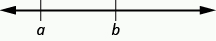$a>b$ is read $a$ is greater than $b$
$a$ is to the right of $b$ on the number line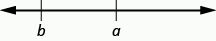The expressions $a<b\text{ and }a>b$ can be read from left-to-right or right-to-left, though in English we usually read from left-to-right. In general,

$a<b\text{ is equivalent to }b>a$.

For example, $7<11\text{ is equivalent to }11>7$.

$a>b\text{ is equivalent to }b<a$.

.For example, $17>4\text{ is equivalent to }4<17$

When we write an inequality symbol with a line under it, such as $a\le b$, it means $a<b$ or $a=b$. We read this $a$ is less than or equal to $b$. Also, if we put a slash through an equal sign, $\ne$, it means not equal.

We summarize the symbols of equality and inequality in the table below.

Algebraic Notation Say
$a=b$ $a$ is equal to $b$
$a\ne b$ $a$ is not equal to $b$
$a<b$ $a$ is less than $b$
$a>b$ $a$ is greater than $b$
$a\le b$ $a$ is less than or equal to $b$
$a\ge b$ $a$ is greater than or equal to $b$

### Symbols $<$ and $>$

The symbols $<$ and $>$ each have a smaller side and a larger side.

smaller side $<$ larger side

larger side $>$ smaller side

The smaller side of the symbol faces the smaller number and the larger faces the larger number.

### Example

Translate from algebra to words:

1. $20\le 35$
2. $11\ne 15 - 3$
3. $9>10\div 2$
4. $x+2<10$

### TRY IT

In the following video we show more examples of how to write inequalities as words.

### Example

The information in the table below compares the fuel economy in miles-per-gallon (mpg) of several cars. Write the appropriate symbol $\text{=},\text{<},\text{ or }\text{>}$ in each expression to compare the fuel economy of the cars.

(credit: modification of work by Bernard Goldbach, Wikimedia Commons)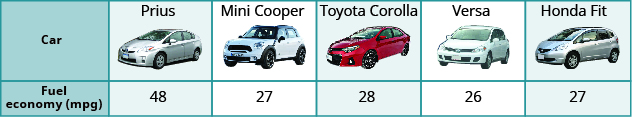1. MPG of Prius_____ MPG of Mini Cooper
2. MPG of Versa_____ MPG of Fit
3. MPG of Mini Cooper_____ MPG of Fit
4. MPG of Corolla_____ MPG of Versa
5. MPG of Corolla_____ MPG of Prius

### TRY IT

Grouping symbols in algebra are much like the commas, colons, and other punctuation marks in written language. They indicate which expressions are to be kept together and separate from other expressions. The table below lists three of the most commonly used grouping symbols in algebra.

Common Grouping Symbols
parentheses ( )
brackets [ ]
braces { }

Here are some examples of expressions that include grouping symbols. We will simplify expressions like these later in this section.

$\begin{array}{cc}8\left(14 - 8\right)21 - 3\\\left[2+4\left(9 - 8\right)\right]\\24\div \left\{13 - 2\left[1\left(6 - 5\right)+4\right]\right\}\end{array}$

## Identify Expressions and Equations

What is the difference in English between a phrase and a sentence? A phrase expresses a single thought that is incomplete by itself, but a sentence makes a complete statement. “Running very fast” is a phrase, but “The football player was running very fast” is a sentence. A sentence has a subject and a verb.

In algebra, we have expressions and equations. An expression is like a phrase. Here are some examples of expressions and how they relate to word phrases:

Expression Words Phrase
$3+5$ $3\text{ plus }5$ the sum of three and five
$n - 1$ $n$ minus one the difference of $n$ and one
$6\cdot 7$ $6\text{ times }7$ the product of six and seven
$\frac{x}{y}$ $x$ divided by $y$ the quotient of $x$ and $y$

Notice that the phrases do not form a complete sentence because the phrase does not have a verb. An equation is two expressions linked with an equal sign. When you read the words the symbols represent in an equation, you have a complete sentence in English. The equal sign gives the verb. Here are some examples of equations:

Equation Sentence
$3+5=8$ The sum of three and five is equal to eight.
$n - 1=14$ $n$ minus one equals fourteen.
$6\cdot 7=42$ The product of six and seven is equal to forty-two.
$x=53$ $x$ is equal to fifty-three.
$y+9=2y - 3$ $y$ plus nine is equal to two $y$ minus three.

### Expressions and Equations

An expression is a number, a variable, or a combination of numbers and variables and operation symbols.
An equation is made up of two expressions connected by an equal sign.

### example

Determine if each is an expression or an equation:

1. $16 - 6=10$
2. $4\cdot 2+1$
3. $x\div 25$
4. $y+8=40$

Solution

 1. $16 - 6=10$ This is an equation—two expressions are connected with an equal sign. 2. $4\cdot 2+1$ This is an expression—no equal sign. 3. $x\div 25$ This is an expression—no equal sign. 4. $y+8=40$ This is an equation—two expressions are connected with an equal sign.

### Learning Outcomes

• Use the order of operations to simplify mathematical expressions
• Simplify mathematical expressions involving addition, subtraction, multiplication, division, and exponents

## Simplify Expressions Containing Exponents

To simplify a numerical expression means to do all the math possible. For example, to simplify $4\cdot 2+1$ we’d first multiply $4\cdot 2$ to get $8$ and then add the $1$ to get $9$. A good habit to develop is to work down the page, writing each step of the process below the previous step. The example just described would look like this:

$4\cdot 2+1$
$8+1$
$9$

Suppose we have the expression $2\cdot 2\cdot 2\cdot 2\cdot 2\cdot 2\cdot 2\cdot 2\cdot 2$. We could write this more compactly using exponential notation. Exponential notation is used in algebra to represent a quantity multiplied by itself several times. We write $2\cdot 2\cdot 2$ as ${2}^{3}$ and $2\cdot 2\cdot 2\cdot 2\cdot 2\cdot 2\cdot 2\cdot 2\cdot 2$ as ${2}^{9}$. In expressions such as ${2}^{3}$, the $2$ is called the base and the $3$ is called the exponent. The exponent tells us how many factors of the base we have to multiply.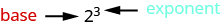$\text{means multiply three factors of 2}$
We say ${2}^{3}$ is in exponential notation and $2\cdot 2\cdot 2$ is in expanded notation.

### Exponential Notation

For any expression ${a}^{n},a$ is a factor multiplied by itself $n$ times if $n$ is a positive integer.

${a}^{n}\text{ means multiply }n\text{ factors of }a$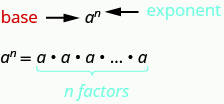The expression ${a}^{n}$ is read $a$ to the ${n}^{th}$ power.

For powers of $n=2$ and $n=3$, we have special names.

$a^2$ is read as “$a$ squared”

$a^3$ is read as “$a$ cubed”

The table below lists some examples of expressions written in exponential notation.

Exponential Notation In Words
${7}^{2}$ $7$ to the second power, or $7$ squared
${5}^{3}$ $5$ to the third power, or $5$ cubed
${9}^{4}$ $9$ to the fourth power
${12}^{5}$ $12$ to the fifth power

### example

Write each expression in exponential form:

1. $16\cdot 16\cdot 16\cdot 16\cdot 16\cdot 16\cdot 16$
2. $\text{9}\cdot \text{9}\cdot \text{9}\cdot \text{9}\cdot \text{9}$
3. $x\cdot x\cdot x\cdot x$
4. $a\cdot a\cdot a\cdot a\cdot a\cdot a\cdot a\cdot a$

### try it

In the video below we show more examples of how to write an expression of repeated multiplication in exponential form.

### example

Write each exponential expression in expanded form:

1. ${8}^{6}$
2. ${x}^{5}$

### try it

To simplify an exponential expression without using a calculator, we write it in expanded form and then multiply the factors.

### example

Simplify: ${3}^{4}$

## Simplify Expressions Using the Order of Operations

We’ve introduced most of the symbols and notation used in algebra, but now we need to clarify the order of operations. Otherwise, expressions may have different meanings, and they may result in different values.

For example, consider the expression:

$4+3\cdot 7$

$\begin{array}{cccc}\hfill \text{Some students say it simplifies to 49.}\hfill & & & \hfill \text{Some students say it simplifies to 25.}\hfill \\ \begin{array}{ccc}& & \hfill 4+3\cdot 7\hfill \\ \text{Since }4+3\text{ gives 7.}\hfill & & \hfill 7\cdot 7\hfill \\ \text{And }7\cdot 7\text{ is 49.}\hfill & & \hfill 49\hfill \end{array}& & & \begin{array}{ccc}& & \hfill 4+3\cdot 7\hfill \\ \text{Since }3\cdot 7\text{ is 21.}\hfill & & \hfill 4+21\hfill \\ \text{And }21+4\text{ makes 25.}\hfill & & \hfill 25\hfill \end{array}\hfill \end{array}$

Imagine the confusion that could result if every problem had several different correct answers. The same expression should give the same result. So mathematicians established some guidelines called the order of operations, which outlines the order in which parts of an expression must be simplified.

### Order of Operations

When simplifying mathematical expressions perform the operations in the following order:
1. Parentheses and other Grouping Symbols

• Simplify all expressions inside the parentheses or other grouping symbols, working on the innermost parentheses first.

2. Exponents

• Simplify all expressions with exponents.

3. Multiplication and Division

• Perform all multiplication and division in order from left to right. These operations have equal priority.

• Perform all addition and subtraction in order from left to right. These operations have equal priority.

Students often ask, “How will I remember the order?” Here is a way to help you remember: Take the first letter of each key word and substitute the silly phrase. Please Excuse My Dear Aunt Sally.

Order of Operations
Excuse Exponents
My Dear Multiplication and Division

It’s good that ‘My Dear’ goes together, as this reminds us that multiplication and division have equal priority. We do not always do multiplication before division or always do division before multiplication. We do them in order from left to right.
Similarly, ‘Aunt Sally’ goes together and so reminds us that addition and subtraction also have equal priority and we do them in order from left to right.

### example

Simplify the expressions:

1. $4+3\cdot 7$
2. $\left(4+3\right)\cdot 7$

Solution:

 1. $4+3\cdot 7$ Are there any parentheses? No. Are there any exponents? No. Is there any multiplication or division? Yes. Multiply first. $4+\color{red}{3\cdot 7}$ Add. $4+21$ $25$
 2. $(4+3)\cdot 7$ Are there any parentheses? Yes. $\color{red}{(4+3)}\cdot 7$ Simplify inside the parentheses. $(7)7$ Are there any exponents? No. Is there any multiplication or division? Yes. Multiply. $49$

### example

Simplify:

1. $\text{18}\div \text{9}\cdot \text{2}$
2. $\text{18}\cdot \text{9}\div \text{2}$

### example

Simplify: $18\div 6+4\left(5 - 2\right)$.

### try it

In the video below we show another example of how to use the order of operations to simplify a mathematical expression.

When there are multiple grouping symbols, we simplify the innermost parentheses first and work outward.

### example

$\text{Simplify: }5+{2}^{3}+3\left[6 - 3\left(4 - 2\right)\right]$.

### try it

In the video below we show another example of how to use the order of operations to simplify an expression that contains exponents and grouping symbols.

### example

Simplify: ${2}^{3}+{3}^{4}\div 3-{5}^{2}$.